# Group

A group of kids wanted to ride. When the children were divided into groups of 3 children, one remain. When divided into groups of 4 children, 1 remains. When divided into groups of 6 children, 1 remains. After divided into groups of 5 children, no one left. How many are there?

n =  25

### Step-by-step explanation: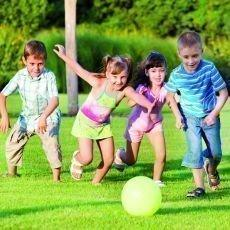Did you find an error or inaccuracy? Feel free to write us. Thank you!Tips to related online calculators
Do you want to calculate least common multiple two or more numbers?
Do you solve Diofant problems and looking for a calculator of Diofant integer equations?

## Related math problems and questions:

• Lesson exercising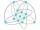The lesson of physical education, pupils are first divided into three groups so that each has the same number. The they redistributed, but into six groups. And again, it was the same number of children in each group. Finally they divided into nine equal g
• Dance groupThe dance group formed groups of 4, 5, and 6 members. Always one dancer remains. How many dancers were there in the whole group?
• Unknown integerFind the smallest integer: divided by 2, the remainder is 1. divided by 3, the remainder is 2. divided by 4, the remainder is 3. ... divided by eight, the remainder is 7, divided by 9 the remainder is 8.
• Two hundred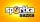Two hundred ten athletes competed in three athletics races on three fields. One hundred five athletes competed in the first, 60 in the second, and everyone else in the third. On the individual courts, the athletes were divided into groups. Each group, alt
• CherriesCherries in the bowl can be divided equally among 3 or 15 or 5 children. How many are the minimum cherries in the bowl?
• Nuts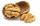How many we must have at least nuts if we can equally divide it to 10 children, 12 children or 15 children and any nut left?
• PlumsIn the bowl are plums. How many would be there if we can divide it equally among 8, 10 and 11 children?
• SchoolLess than 500 pupils attend school. When it is sorted into pairs, one pupil remains. Similarly, when sorted into 3, 4, 5 and 6 members team one remains. Sorted to seven members teams, no left behind. How many pupils are attending this school?
• Balls groups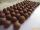Karel pulled the balls out of his pocket and divided them into groups. He could divide them in four, six, or seven, and no ball ever left. How little could be a ball?
• Dividing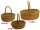When dividing the tangerines into packages of 8 or 10, one always remained. How many were there if there were more than 250 and less than 300?
• LCMWhat is the least common multiple of 5, 50, 14?
• DivisibilityDetermine the smallest integer which divided 11 gives remainder 4 when divided 15 gives remainder 10 and when divided by 19 gives remainder 16.
• Parachutists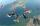Parachutists during freefall firstly held in groups of 4, then of 6, then 9, 12 and finally of 18 members. How many parachutists jump at least should be, if at each group must all be involved.
• The smallest numberWhat is the smallest number that can be divided by both 5 and 7
• GroupsIn the 6th class there are 60 girls and 72 boys. We want to divide them into groups so that the number of girls and boys is the same. How many groups can you create? How many girls will be in the group?
• Two groupsThe group of 10 girls should be divided into two groups with at least 4 girls in each group. How many ways can this be done?
• Athletics teamAll athletes in the athletics team from Ostrava can start in four, five, six and seven stages, and no one will be present. How many average athletes are in one athletic group if there are a total of twelve groups in the section? Consider the smallest numb